Latest Banking jobs   »

# Quantitative Aptitude Quiz For IBPS PO Prelims 2022- 18th September

Directions (1-5): Read the following table carefully and answer the questions given below. Table shows average number of cheese packets (Feta Cheese Packets + Mozzarella Cheese Packets) and ratio of feta cheese packets to mozzarella cheese packets sold by four different sellers.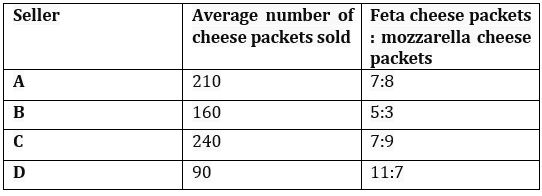Q1. Find the ratio of number of feta cheese packets sold by B to number of mozzarella cheese packets sold by A.
(a) 25 :29
(b) 21 :28
(c) 22 :25
(d) 25 :28
(e) 25 :23

Q2. If D sold each feta cheese packet at Rs.60 & each mozzarella cheese packet at Rs.75, then find the total earning (in Rs.) of D by selling all the cheese packet.
(a) Rs.11450
(b) Rs.11210
(c) Rs.11780
(d) Rs.11650
(e) Rs.11850

Q3. Find the difference between number of mozzarella cheese packets sold by C and number of feta cheese packets sold by B.
(a) 65
(b) 60
(c) 105
(d) 70
(e) 90

Q4. If E sold 40% more feta cheese packets than that by B and mozzarella cheese packets sold by E is 45 less than that by C, then find total number of cheese packet (mozzarella + feta) sold by E.
(a) 505
(b) 485
(c) 460
(d) 580
(e) 620

Q5. Number of mozzarella cheese packets sold by A is how much percent more/less than number of feta cheese packets sold by B?
(a) 10%
(b) 12%
(c) 6%
(d) 18%
(e) 20%

Directions (6-10): In each of these questions, two equations (I) and (II) are given. You have to solve both the equations and give answer
(a) if x>y
(b) if x≥y
(c) if x<y
(d) if x ≤y
(e) if x = y or no relation can be established between x and y

Q6. I. (x-2)²-4=0
II. y²+1-2y=0

Q7. I. 3x+2y=5
II. 4x+6y=10

Q8. I. 9x² – 54x + 77 = 0
II. 12y² – 55y + 63 = 0

Q9. I. (x – 1) ² = 121
II. y² – 24y + 144 = 0

Q10.I. 7x² – 23x + 6 = 0
II. y² – 7y + 12 = 0

Directions (11-15): Read the following data carefully and answer the questions given below. Data shows all the students from two different college, students joined either gym or yoga. No students from any of the given colleges joined both the places.
Number of students joined gym from college N is 80. Ratio of number of students joined gym from college M and joined yoga from college N is 7:5 respectively. Total number of students from college M is 149. Number of students joined yoga from college M is 55% of number of students joined gym from college N.

Q11. Number of students joined gym from college M is what percent more/less than number of students joined yoga from college N?
(a) 60%
(b) 55%
(c) 50%
(d) 40%
(e) 30%

Q12. Find the ratio between number of students joined gym from college N to number of students joined yoga from college M.
(a) 19:11
(b) 18:19
(c) 22:15
(d) 20:19
(e) 20:11

Q13. Ratio of number of boys to girls joined gym from college N and M is 5:3 and 8:7 respectively. Find the difference between number of boys joined gym from college M and N together to that of girls from same colleges.
(a) 22
(b) 25
(c) 18
(d) 27
(e) 12

Q14. Find the difference between total number of students joined gym and total number of students joined yoga from both the colleges.
(a) 62
(b) 70
(c) 54
(d) 58
(e) 66

Q15. Number of students joined yoga from college P is 25% more than that from college M. Number of students joined yoga from college N is approximately what percentage of that from college P?
(a) 132%
(b) 128%
(c) 125%
(d) 136%
(e) 140%

Solutions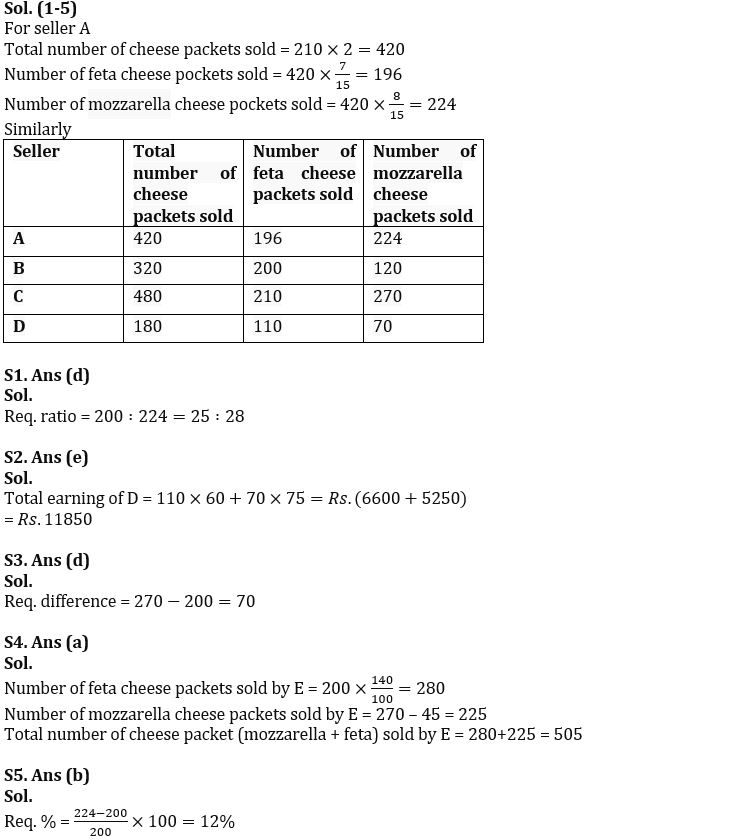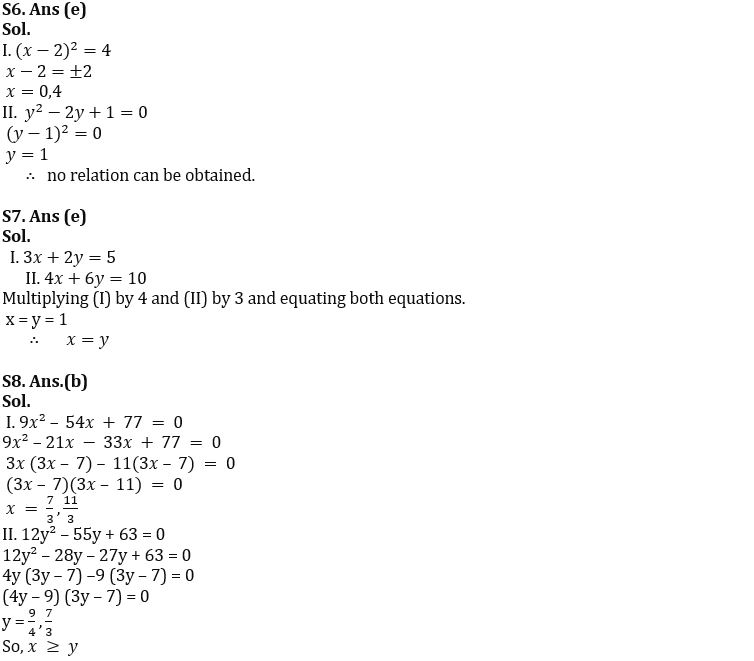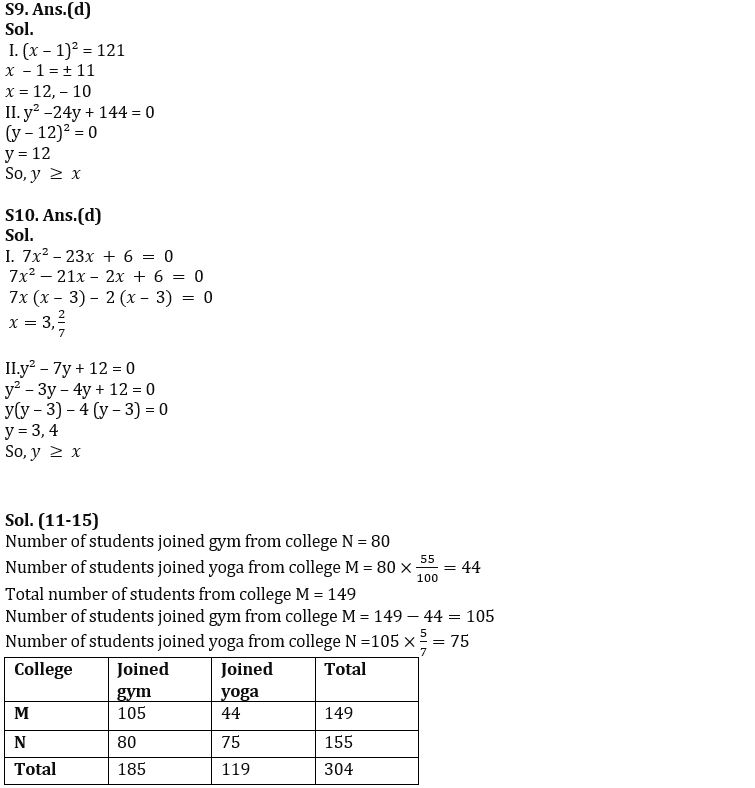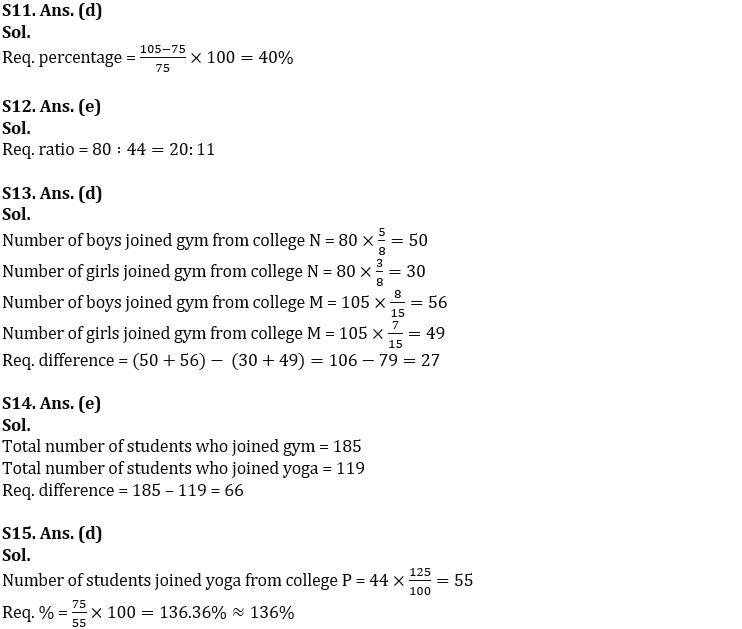#### Congratulations!Download Hindu Review of October 2021: Free PDF